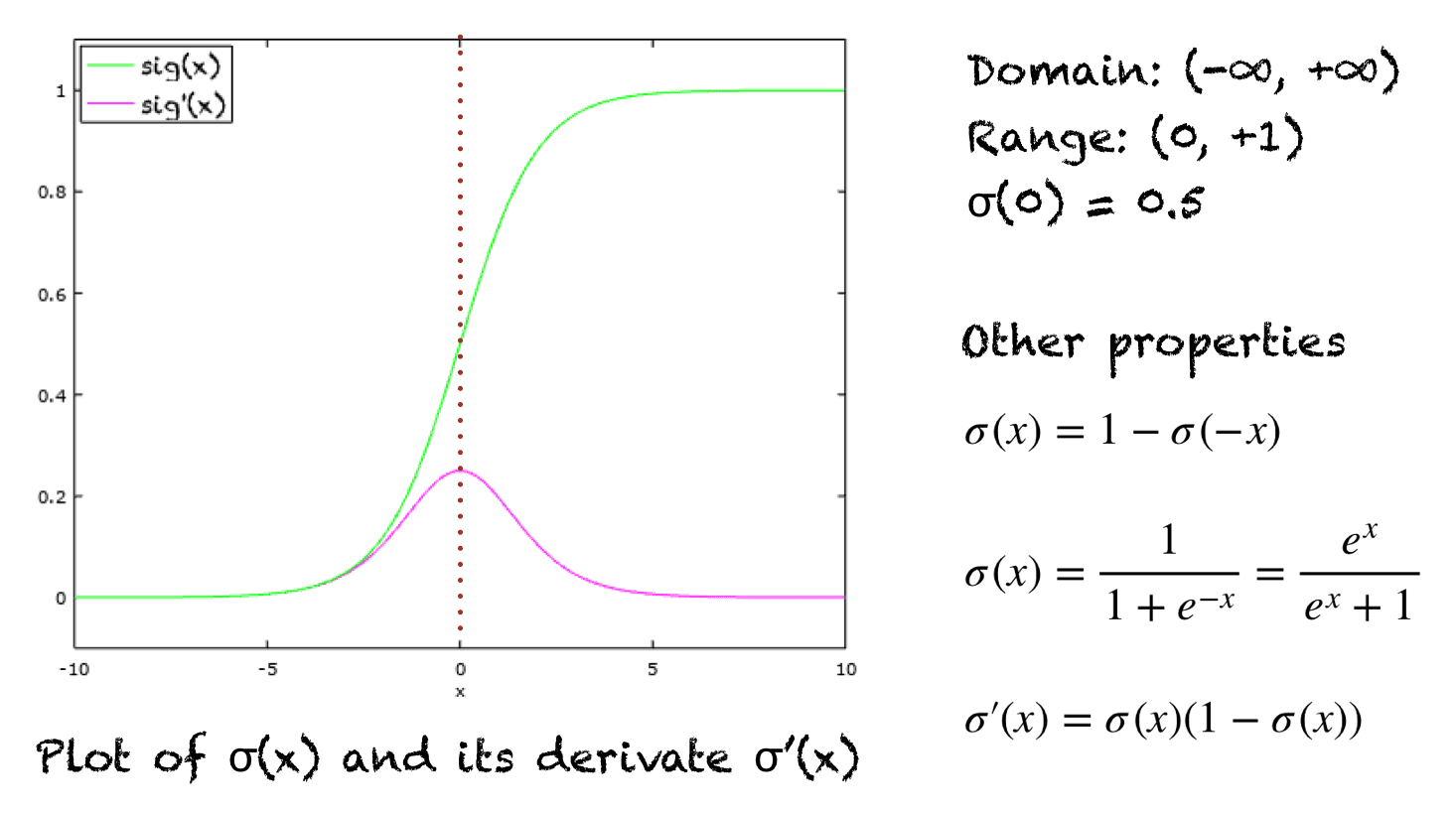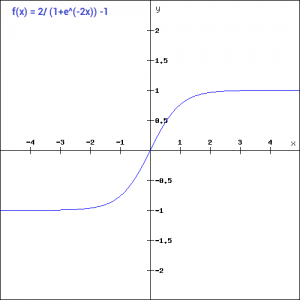# Activation Function: Sigmoid## What is an activation function?Activation functions get their names from being used in neural networks as they decide whether a particular neuron should be activated. In the context of this release, the sigmoid function will be discussed in detail as it is used in the logistic regression algorithm for binary classification.

### Sigmoid Activation function

The sigmoid function’s main use is to limit the input values and get them between 0 and 1.

The formula for the sigmoid function is:

This function looks like an “S” when plotted on the 2-D graph.

Logistic regression inherently is a modification of linear regression, and the sigmoid function is used to convert the linear equation viable for classification. The final classification is made based on the value of σ (sigmoid).

Here y is the output of logistic regression.

This is a continuously increasing function that is differentiable everywhere.

The differentiability is useful in the case of neural networks because during backpropagation, using gradient descent to calculate weights of the neural network derivative of the activation function is taken.

(Some terms might not be clear right now but will be understood after reading the neural networks release)

Unfortunately, this is computationally expensive and not zero-centered so avoided in neural networks and essentially used in binary classification.Python Implementation of the sigmoid function:

This activation function is also called a squashing function because it can take very large values and squash them in the range (0,1).

The sigmoid function is very important in the world of neural networks because if only linear functions were used, then the model would learn linearly. By adding a hidden sigmoid layer, it can work with non-linear problems.

As aforementioned, sigmoid works with only binary classification problems a modified version called softmax is used for multi-class classification situations.

#### Softmax:

This is a function that uses multiple sigmoids in one function. Mathematically it looks like:

Applying softmax gives the probability for the datapoint belonging to each class and its sum is always one.

Python Implementation for softmax function:

 def softmax_function(x): z = np.exp(x) z_ = z/z.sum() return z_

#### Tanh:

Tanh is also an activation function. This function modifies the sigmoid by making it symmetric around the origin.

The formula for Tanh is:

tanh(z)=2sigmoid(2z)-1

Or

tanh(x) = 2/(1+e^(-2x)) -1If you notice, the graph for both sigmoid and tanh is an “S” shape, but tanh is centered around the origin and keeps its range between -1 and 1, unlike sigmoid’s 0 to 1 range.

Python implementation for tanh:

 def tanh(x): z = (2/(1 + np.exp(-2*x))) -1 return z

#### Swish:

Swish is another sigmoid modification developed by researchers at Google while looking for a computationally efficient function.

Formula for swish is:

f(x) = x*sigmoid(x)

or

f(x) = x/(1-e^-x)

Python implementation for swish:

 def swish_function(x): return x/(1-np.exp(-x))

This function was created to outperform ReLU (another activation function). This is the version of sigmoid, where values vary from negative infinity to infinity.

In conclusion, the sigmoid function and its variations are mostly used for classification problems.

The sigmoid activation function is a commonly used non-linear activation function in artificial neural networks. It is also known as the logistic function due to its mathematical form resembling the logistic curve. The sigmoid function maps the input values to a range between 0 and 1, which makes it suitable for problems that require binary classification or probabilistic outputs.

### The sigmoid function is defined as:

σ(x) = 1 / (1 + e^(-x))

where x is the input to the function and e is the base of the natural logarithm (approximately 2.71828).

The sigmoid function has several important properties that make it useful in neural networks:

1. Non-linearity: The sigmoid function introduces non-linearity to the network, allowing it to model complex relationships between inputs and outputs. The non-linear nature of the sigmoid function enables neural networks to approximate non-linear functions and solve more complex tasks.
2. Differentiability: The sigmoid function is differentiable for all values of x. This property is crucial for training neural networks using gradient-based optimization algorithms, such as backpropagation. The ability to calculate derivatives allows the network to update its weights and learn from the training data.
3. Output Range: The sigmoid function maps the input values to a range between 0 and 1. This property makes it suitable for binary classification tasks, where the network needs to assign probabilities to the two classes. The output value of the sigmoid function can be interpreted as the probability of the input belonging to the positive class.

### Despite its usefulness, the sigmoid activation function has some limitations:

1. Vanishing Gradient Problem: The gradient of the sigmoid function becomes very small for large positive and negative values of x. This causes the gradients to “vanish” during backpropagation, which can slow down or hinder the learning process in deep neural networks. The vanishing gradient problem can make it difficult for the network to propagate errors and update the weights effectively.
2. Output Saturation: The output of the sigmoid function saturates at 0 for large negative values of x and at 1 for large positive values of x. When the network is in these saturated regions, the gradients become very small, and the network may struggle to make further progress in learning. This can result in slower convergence or even the complete cessation of learning.
3. Not Zero-Centered: The sigmoid function is not zero-centered, meaning that its output values are always positive. This can introduce biases in the subsequent layers of the network, particularly when combined with certain weight initialization techniques. Biases in the network can affect its ability to learn and generalize effectively.
4. Computationally Expensive: The exponential calculations involved in evaluating the sigmoid function can be computationally expensive, especially when dealing with large matrices or in deep neural networks. This can lead to slower training and inference times compared to other activation functions.

Despite its limitations, the sigmoid activation function still finds applications in certain scenarios, such as binary classification tasks and as an activation function in the output layer for probabilistic outputs. However, for hidden layers in deep neural networks, other activation functions like ReLU (Rectified Linear Unit) or variants such as Leaky ReLU, ELU (Exponential Linear Unit), or SELU (Scaled Exponential Linear Unit) are often preferred due to their ability to alleviate the vanishing gradient problem and improve training performance.

In conclusion, the sigmoid activation function is a non-linear function commonly used in artificial neural networks. It maps input values to a range between 0 and 1 and is useful for binary classification tasks and probabilistic outputs. However, it suffers from the vanishing gradient problem, output saturation, lack of zero-centering, and computational complexity. As a result, other activation functions are often preferred in the hidden layers of deep neural networks.